Test: Analog Electronics - 4

# Test: Analog Electronics - 4

Test Description

## 25 Questions MCQ Test Analog Circuits | Test: Analog Electronics - 4

Test: Analog Electronics - 4 for Electrical Engineering (EE) 2022 is part of Analog Circuits preparation. The Test: Analog Electronics - 4 questions and answers have been prepared according to the Electrical Engineering (EE) exam syllabus.The Test: Analog Electronics - 4 MCQs are made for Electrical Engineering (EE) 2022 Exam. Find important definitions, questions, notes, meanings, examples, exercises, MCQs and online tests for Test: Analog Electronics - 4 below.
Solutions of Test: Analog Electronics - 4 questions in English are available as part of our Analog Circuits for Electrical Engineering (EE) & Test: Analog Electronics - 4 solutions in Hindi for Analog Circuits course. Download more important topics, notes, lectures and mock test series for Electrical Engineering (EE) Exam by signing up for free. Attempt Test: Analog Electronics - 4 | 25 questions in 25 minutes | Mock test for Electrical Engineering (EE) preparation | Free important questions MCQ to study Analog Circuits for Electrical Engineering (EE) Exam | Download free PDF with solutions
 1 Crore+ students have signed up on EduRev. Have you?
Test: Analog Electronics - 4 - Question 1

### If the Q of a single stage single tuned amplifier is doubled, the bandwidth will

Detailed Solution for Test: Analog Electronics - 4 - Question 1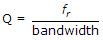. If Q is doubles, bandwidth becomes half.

Test: Analog Electronics - 4 - Question 2

### In full wave rectification, if the input frequency is 50 Hz, then frequency at the output of filter is

Detailed Solution for Test: Analog Electronics - 4 - Question 2

Filter output will give d.c. signal and d.c. signal Passed zero frequency.

Test: Analog Electronics - 4 - Question 3

### The circuit in the given figure is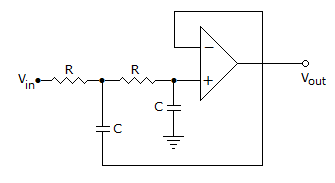Detailed Solution for Test: Analog Electronics - 4 - Question 3

It is a L.P.F. of second order.

Test: Analog Electronics - 4 - Question 4

The turn ratio of a transformer is 20:1, if a load of 10Ω is connected across the secondary, what will be the effective resistance seen looking into the Primary?

Detailed Solution for Test: Analog Electronics - 4 - Question 4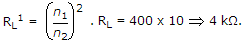Test: Analog Electronics - 4 - Question 5

In a CE amplifier circuit the ac voltage between emitter and ground

Detailed Solution for Test: Analog Electronics - 4 - Question 5

Common emitter means emitter at ground potential.

Test: Analog Electronics - 4 - Question 6

A 120 V, 30 Hz source feeds a half wave rectifier circuit through a 4 : 1 step down transformer, the average output voltage is

Detailed Solution for Test: Analog Electronics - 4 - Question 6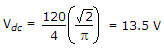.

Test: Analog Electronics - 4 - Question 7

The slew rate of an ideal op-amp is

Detailed Solution for Test: Analog Electronics - 4 - Question 7

Slew rate signifies the fastest change which can occurs in output voltage. In an ideal op-amp the output can change instantaneously.

Test: Analog Electronics - 4 - Question 8

Regulation of the DC power supply of 12 V, 100 mA is the effective resistance of power supply is 20 Ω

Detailed Solution for Test: Analog Electronics - 4 - Question 8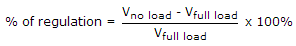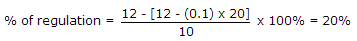.

Test: Analog Electronics - 4 - Question 9

A bridge diode circuit using ideal diodes has in input voltage of 20 sin ωt volts. The average and rms values of output voltage are

Detailed Solution for Test: Analog Electronics - 4 - Question 9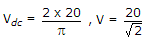.

Test: Analog Electronics - 4 - Question 10

In the circuit of figure v1 = 8 V and v2 = 0. Which diode will conduct (Assume ideal diodes)?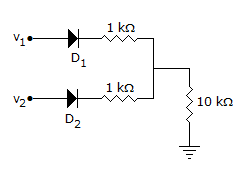Detailed Solution for Test: Analog Electronics - 4 - Question 10

Since only v1 is positive and more than 0.7 V only D1 will conduct.

Test: Analog Electronics - 4 - Question 11

Assertion (A): An op-amp has high voltage gain, high input impedance and low output impedance

Reason (R): Negative feedback increases output impedance

Detailed Solution for Test: Analog Electronics - 4 - Question 11

Negative feedback reduces output impedance.

Test: Analog Electronics - 4 - Question 12

A transistor has a maximum power dissipation of 350 mW at an ambient temperature of 25ºC. If derating factor is 2 mW/ºC, the maximum power dissipation for 40ºC ambient temperature is

Detailed Solution for Test: Analog Electronics - 4 - Question 12

Derating improves reliability but does not affect maximum power dissipation.

Test: Analog Electronics - 4 - Question 13

The output voltage waveform of a CE amplifier is fed to a dc coupled CRO. The trace on the screen will be

Detailed Solution for Test: Analog Electronics - 4 - Question 13

Due to direct coupling between output and CRO both dc and ac output voltage will appear on screen of CRO.

Test: Analog Electronics - 4 - Question 14

In an RC phase shift oscillator, the total phase shift of the three RC lead networks is

Detailed Solution for Test: Analog Electronics - 4 - Question 14

Each RC network gives 60º phase shift.

Test: Analog Electronics - 4 - Question 15

In the circuit of figure β = 100 and quiescent value of base current is 20 μA. The quiescent value of collector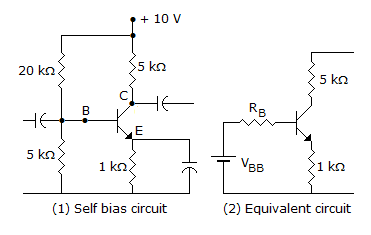current is

Detailed Solution for Test: Analog Electronics - 4 - Question 15

IC = βIB = 2 mA.

Test: Analog Electronics - 4 - Question 16

Values of VT at 20ºC for an ideal P-N diode

Detailed Solution for Test: Analog Electronics - 4 - Question 16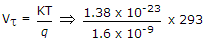.

Test: Analog Electronics - 4 - Question 17

Assertion (A): In an op-amp the voltage gain and band width can be adjusted as per requirement

Reason (R): Large value capacitor can also be fabricated on a chip

Detailed Solution for Test: Analog Electronics - 4 - Question 17

Large value capacitor cannot be fabricated on a chip.

Test: Analog Electronics - 4 - Question 18

In figure which diode will conduct and what will be the value of V0?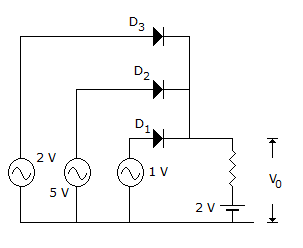Detailed Solution for Test: Analog Electronics - 4 - Question 18

Since battery connected to anode of D, is of 5 V it is forward biased. D1 and D3 are reverse biased. Output voltage is 5 V.

Test: Analog Electronics - 4 - Question 19

For the amplifier circuit of figure, the h parameters of transistor are hib = 25 Ω, hfb = 0.999, hob = 10-6 Ω. The voltage gain is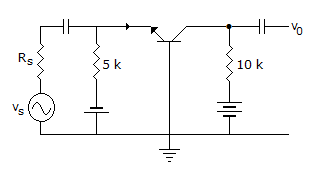Detailed Solution for Test: Analog Electronics - 4 - Question 19

It is a common base circuit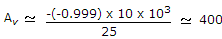.

Test: Analog Electronics - 4 - Question 20

In figure, VCC = + 30 V, R1 = 200 kΩ and R2 = 100 kΩ. If VBE = 0.7 V, the voltage a cross RE =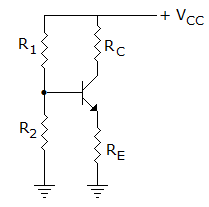Detailed Solution for Test: Analog Electronics - 4 - Question 20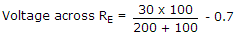.

Test: Analog Electronics - 4 - Question 21

A negative feedback can be of

Detailed Solution for Test: Analog Electronics - 4 - Question 21

Series voltage, series current, shunt voltage and shunt current.

Test: Analog Electronics - 4 - Question 22

In a class C power amplifier the input frequency of ac signal is 1 MHz. If tank circuit has C = 1000 pF, the value of L =

Detailed Solution for Test: Analog Electronics - 4 - Question 22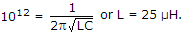Test: Analog Electronics - 4 - Question 23

The Vo of the op-amp circuit shown in the given is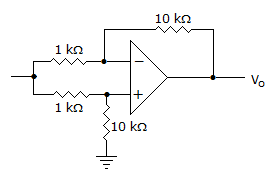Detailed Solution for Test: Analog Electronics - 4 - Question 23

V0 =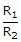(Vs2 - Vs1)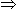[Vs1 - Vs1] = 0.

Test: Analog Electronics - 4 - Question 24

In calculating output impedance of an amplifier the source is replaced by an open circuit.

Detailed Solution for Test: Analog Electronics - 4 - Question 24

It is replaced by short circuit.

Test: Analog Electronics - 4 - Question 25

For transistor 2 N 338 the manufacturer specifies Pmax = 100 mW at 250ºC free air temperature and maximum junction temperature of 125ºC. Its thermal resistance is

Detailed Solution for Test: Analog Electronics - 4 - Question 25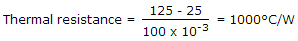.

## Analog Circuits

5 videos|20 docs|22 tests
 Use Code STAYHOME200 and get INR 200 additional OFF Use Coupon Code
Information about Test: Analog Electronics - 4 Page
In this test you can find the Exam questions for Test: Analog Electronics - 4 solved & explained in the simplest way possible. Besides giving Questions and answers for Test: Analog Electronics - 4, EduRev gives you an ample number of Online tests for practice

## Analog Circuits

5 videos|20 docs|22 tests